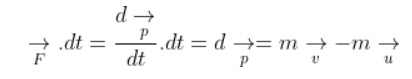# A woman throws an object of mass 500 g`
Question:

A woman throws an object of mass 500 g with a speed of 25 m/s.

(a) what is the impulse imparted to the object?

(b) if the object hits a wall and rebounds with half the original speed, what is the change in momentum of the object?

Solution:

(a) Mass of the object, m = 500 g = 0.5 kg

u = 0

v = 25 m/s

Impulse is given as:Substituting the values, we get impulse = 12.5 N.s

b) m = 0.5 kg

u = +25 m/s

v = -25/2 m/s

∆p = m(v – u) = -18.75 N.s